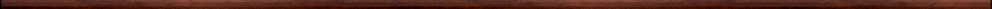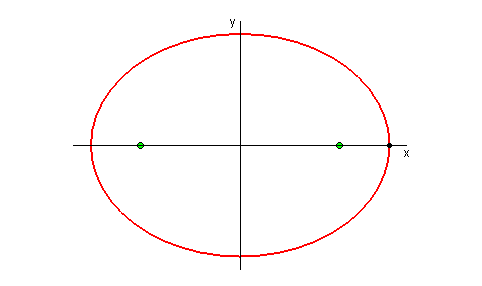Maple worksheets on conic sectionsPrecalculus topics:

They are all compatible with Classic Worksheet Maple 10.The parabola  - parabola.mws

• The geometrical description of a parabola.
• The distance formula.
• The equation of a parabola.

The ellipse  - ellipse.mws

• The geometrical description of an ellipse.
• The equation of an ellipse.

The hyperbola  - hyperbola.mws

• The geometrical description of a hyperbola.
• The equation of an hyperbola.

Translations of standard ellipses and hyperbolas  - translate.mws

• A circle is a limiting case of an ellipse.
• Translating a circle so that its centre moves away from the origin.
• Translating an ellipse so that its centre moves away from the origin.
• Translating a hyperbola so that its centre moves away from the origin.

Rectangular hyperbolas with the coordinate axes as asymptotes  - rotate.mws

• Rotating a rectangular hyperbola about the origin.
• An example.

Top of page

Main index